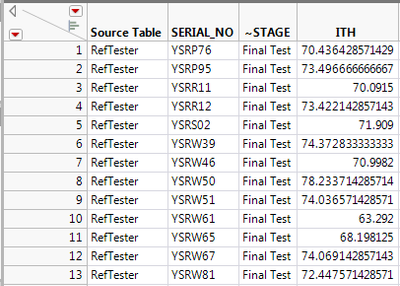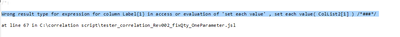Choose Language Hide Translation Bar
Highlighted

## New Column : Set Initial Value from List Data

Hi

The script will read numeric column name from the file. If there is only 1 column, I want to create the new column and assign value in the column with the header reading from file.  In below case, the new column should have initial value = ITHI use below script but it doesn't assign the initial value. Not sure why.

``````ColList2 = Data Table( "raw data" ) << get column names( numeric );

if(n items (ColList2) ==1,
new column("Label",character, nominal,set each value (ColList2));
);

``````

1 ACCEPTED SOLUTION

Accepted Solutions
Highlighted

## Re: New Column : Set Initial Value from List Data

Hi,

There was just one piece missing from the solution: you need to specify the format of the content for ColList2 when using the Get Column Names command (see below)

``````Names default to Here (1);

dt = Current Data table ();

ColList2 = dt << get column names( numeric, string );

if(n items (ColList2) ==1,
dt << new column("TEST", character);
dt:TEST << set each value (ColList2 )
);``````
Thierry R. Sornasse
4 REPLIES 4
Highlighted

## Re: New Column : Set Initial Value from List Data

ColList2 is a list, so you need to indicate which element of the list you want to use when you "set each value".  Since there is only 1 item in the list, you would specify it as

``colList2``
``````ColList2 = Data Table( "raw data" ) << get column names( numeric );

if(n items (ColList2) ==1,
new column("Label",character, nominal,set each value (ColList2));
);``````
Jim
Highlighted

## Re: New Column : Set Initial Value from List Data

try that but it didn't work. Below is error message.Highlighted

## Re: New Column : Set Initial Value from List Data

Ah, I missed the point, that when you get the column names, your are returning them as expressions, not as character strings.  Therefore, change the line of code to:

``new column("Label",character, nominal,set each value (char(ColList2)));``
Jim
Highlighted

## Re: New Column : Set Initial Value from List Data

Hi,

There was just one piece missing from the solution: you need to specify the format of the content for ColList2 when using the Get Column Names command (see below)

``````Names default to Here (1);

dt = Current Data table ();

ColList2 = dt << get column names( numeric, string );

if(n items (ColList2) ==1,
dt << new column("TEST", character);
dt:TEST << set each value (ColList2 )
);``````
Thierry R. Sornasse
Article Labels